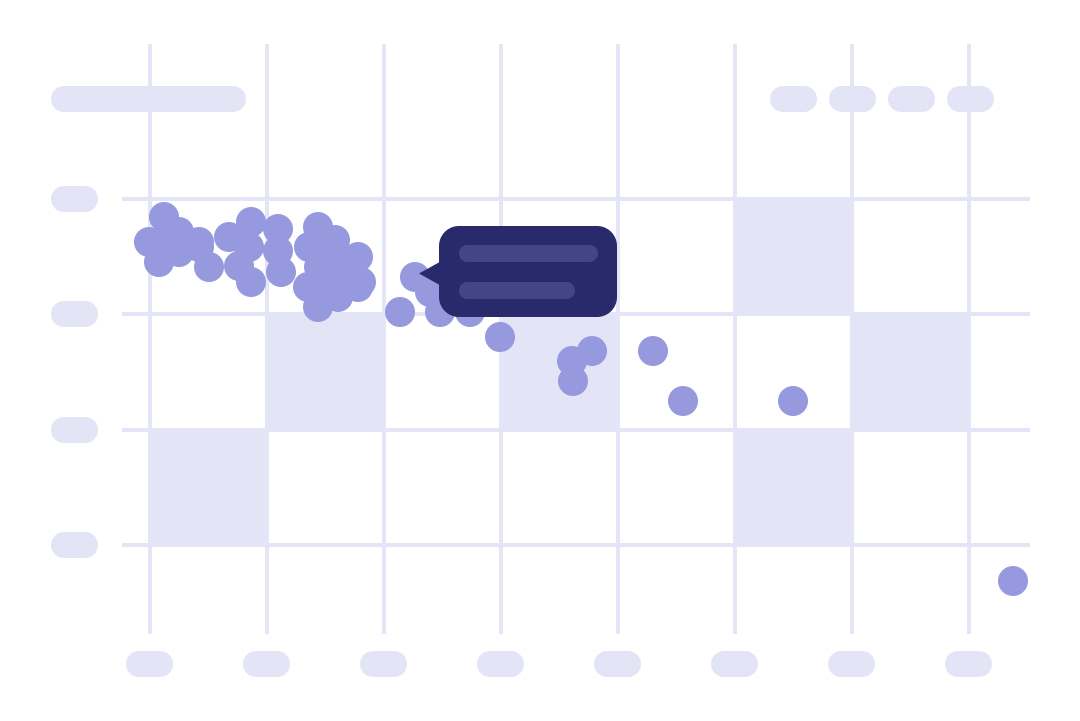Data Science

# How to Create Interactive Visualisations in Colab with Holistic AI and Plotly

June 27, 2023

Visualising data is crucial in any analysis. It facilitates an intuitive understanding of the numbers, allowing us to identify patterns, discrepancies and trends. In machine learning, this serves a particuarly useful purpose. A visual representation of data can be a catalyst for informed decision-making, which can assist in ridding AI systems of damaging biases that impact both their efficacy and fairness.

This effect can be achieved by building an interactive bias measuring and mitigation plot in Python, using the Holistic AI, sklearn and Plotly libraries. This implementation doesn’t need local installations. All steps will be constructed in Google Colab.

The Holistic AI library is an open-source tool to assess and improve the trustworthiness of AI systems. The current version of the library offers a set of techniques to easily measure and mitigate bias across a variety of tasks.

### Imports and data

To get started building the interactive bias measuring and mitigation plots, we first must import the necessary libraries and data. We will be using the Holistic AI Library to implement a set of bias-mitigation techniques, while the sklearn and Plotly libraries will be used for training/testing our machine learning models and creating interactive visualisations respectively. To demonstrate the process, we will be using a data set which centres on the law school bar pass rates of white and non-white students, with protected attributes of race and gender. We pay special attention to race in this case, as preliminary exploration hints at strong inequality in this sensitive attribute.

``````
# install holisticai library
!pip install -q holisticai# import data and preprocessing tools
from sklearn.preprocessing import StandardScaler
from sklearn.model_selection import train_test_split

# simple preprocessing before training.
df_enc = df.copy()
df_enc['bar'] = df_enc['bar'].replace({'FALSE':0, 'TRUE':1})

# split features (X) and target(y), then train test split
X = df_enc.drop(columns=['bar', 'ugpagt3'])
y = df_enc['bar']
X_train, X_test, y_train, y_test = train_test_split(X, y, test_size=0.3, random_state=42)

# StandarScaler transformation
scaler = StandardScaler()
X_train_t = scaler.fit_transform(X_train.drop(columns=['race1', 'gender']))
X_test_t = scaler.transform(X_test.drop(columns=['race1', 'gender']))
```
```

### Bias metrics and accuracy

In our interactive visualisation, we will cover bias metrics and accuracy. When building machine learning models, it is important to assess their accuracy, defined as a measure of how well the model performs on the data on which it is trained. However, accuracy alone is not enough to determine the trustworthiness of a machine learning model. We also need to assess whether the model is biased and if that bias is leading to the unfair treatment of certain groups of people – in our example, non-white applicants to law school.

The code below details how these metrics can be applied.

``````
# import model and metrics
from holisticai.bias.metrics import disparate_impact
from sklearn.metrics import roc_auc_score
from sklearn.ensemble import RandomForestClassifier
from sklearn.metrics import RocCurveDisplay

# create empty lists to store bias metrics and accuracy
bias_metrics = []
loss_curve = []

# create a loop to train the model i times
for i in range(1,100):

# random forest with i estimators
model = RandomForestClassifier(n_estimators=i)

# fit model
model.fit(X_train_t, y_train)

# make predictions
y_pred = model.predict(X_test_t)

# set up groups, prediction array and true (aka target/label) array.
group_a = X_test["race1"]=='non-white'  # non-white vector
group_b = X_test["race1"]=='white'      # white vector
y_true  = y_test                        # true vector

# create a table with classification bias metrics and accuracy
loss_curve.append(roc_auc_score(y_true, y_pred))
bias_metrics.append(disparate_impact(group_a, group_b, y_pred))
```
```

### Create interactive visualisations

In the penultimate step, we will create interactive plots to visualise the bias metrics and accuracy of our machine learning model. In this context, we can see the correlation between these two metrics. How does the accuracy-bias trade-off change with variations in model parameters? The figure illustrates this relationship by showing how the disparate impact changes as the number of estimators of a ‘random forest’ model – a machine learning algorithm combining multiple decision trees – increase.

``````
# import libraries to data manipulation and visualization
import pandas as pd
import plotly.express as px

# concat bias and accuracy metrics
df = pd.concat([pd.DataFrame(bias_metrics), pd.DataFrame(loss_curve)], axis = 1)
df.columns = ['Bias Curve', 'Loss Curve']

# create a scatter plot with bias curve and loss curve
fig = px.scatter(df,
y = 'Bias Curve',
x = 'Loss Curve',
template = 'plotly_white',
title = 'Bias vs Accuracy')

# update marker size
fig.update_traces(marker_size = 15)

# change figure size
fig.update_layout(
height=500,
width=900)

# see the result
fig.show()
```
```

As the finished product above shows, in this article we have demonstrated how to build interactive bias measuring and mitigation plots in Python using the Holistic AI, sklearn and Plotly libraries. By creating simple visualisations and presenting the data in an engaging manner, we can better understand the results of the bias mitigation techniques used and gain essential insights into the performance of our machine learning models.

While we focused on a specific data set in this example, you can use different configurations to suit your needs. To see the interactive graph in action, access the code via this Colab link.

Discover additional methods for assessing and addressing bias by exploring the Holistic AI Library, an open-source tool aimed at enhancing the trustworthiness and transparency of AI systems.

Written by Kleyton da Costa, Researcher at Holistic AI.

DISCLAIMER: This blog article is for informational purposes only. This blog article is not intended to, and does not, provide legal advice or a legal opinion. It is not a do-it-yourself guide to resolving legal issues or handling litigation. This blog article is not a substitute for experienced legal counsel and does not provide legal advice regarding any situation or employer.

## Manage risks. Embrace AI.

Our AI Governance, Risk and Compliance platform empowers your enterprise to confidently embrace AI